SYLLABUS  Previous: 5.4 A scheme for  Up: 5 MONTE-CARLO METHOD  Next: 5.6 Computer quiz

## 5.5 When should you use Monte Carlo methods?

Slide : [ high dimensions - complex BC - parallelization || VIDEO login]

Particle methods generally are efficient for problems dealing with a large number of dimensions and complex geometries: table 5.5.0#tab.1 suggests that Monte Carlo methods become more efficient than FD or FEM when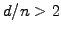(in the limit of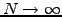and neglecting computer hardware issues).

Table 5.5.0#tab.1: Accuracy for a problem with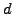-dimensions.
Method Accuracy
Monte Carlo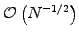,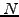is the number of particles.
FEM/FD (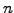:th-order)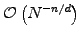,is number of grid points.

The MC method is also well suited for problems with complicated boundary conditions. Imagine for example a 3D cube with a bouncing ball inside. Let the cube contain a non collisional gas, so that the atoms bounce back from the surfaces of the cube and the ball. For simplicity let the cube and the ball have infinite mass during the collisions with the gas particles. The distribution of the gas atoms is fairly easy to compute with the MC method, but nearly untraceable with a fluid method.

Parallelization is easy and efficient if the problem is linear and the particles are decoupled: just run a copy of the simulation program on several machines simultaneously. The final result is obtained by summation and normalization of the results from the individual machines, since the Monte Carlo time stepping is a diagonal linear operation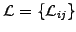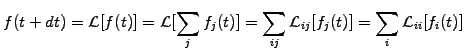(1)

where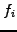is either the particle or its projection (1.4.7#eq.3). Be careful, however, to seed the random number generators differently on the each machines, or the simulations will all be identical!

Unfortunately, the MC method is not that easy to parallelize for non-linear problems: ifand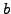are functions of the density distribution, the continuous density distribution function needs to be approximated after each step. This will dramatically reduce the performance on a parallel machine.

SYLLABUS  Previous: 5.4 A scheme for  Up: 5 MONTE-CARLO METHOD  Next: 5.6 Computer quiz